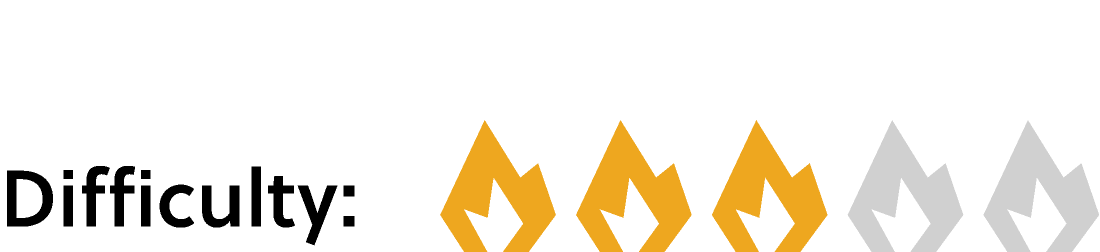## It's A Face Off

It's natural to focus on the surfaces, or faces, of a $3\text{D}$ shape like a cube, but it also has two other kinds of parts. The faces meet at edges, and the edges meet at vertices.

We can't always explode a shape to count its parts, though. If we know that a cube has six square faces, are there other ways to count its other parts? Keep reading to see one way we can do this, or skip ahead to the challenge if you feel ready for a tougher task.

In situations like these, a logical, systematic method can help us avoid undercounting or overcounting. Let's think about how all the parts of the cube are connected. If we can relate the edges and vertices to the six faces, it might give us clues about how best to count them.

Each face is a square. A square has four sides, so six squares have $6 \times 4 = 24$ sides in all. If we were to make a cube from six squares, we would need to glue the squares' sides together. The glued-together sides form the edges of the square, and every edge is shared by two square faces. This means the cube will have $\frac{6 \times 4}{2} = 12$ edges.

We can use similar reasoning to count the cube's vertices. Every square has four vertices, but now every vertex is shared by three faces. So, the cube has $\frac{6 \times 4}{3} = 8$ vertices.

We could also have reasoned that each of the cube's twelve edges has two vertices at its ends, and when the cube is glued together, each vertex is shared by three edges. This way of counting vertices also gives us $\frac{12 \times 2}{3} = 8$ vertices.

By reasoning about how the parts of other $3\text{D}$ shapes are connected and using some careful counting techniques, we can determine how many of each part the shape has. Try it on the challenge below.

# Today's Challenge

On the left is a cube and on the right is a tetrahedron. Initially, the cube has more faces.

Which has more faces after being transformed? $\\[1em]$